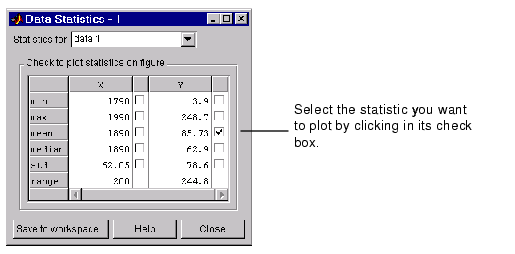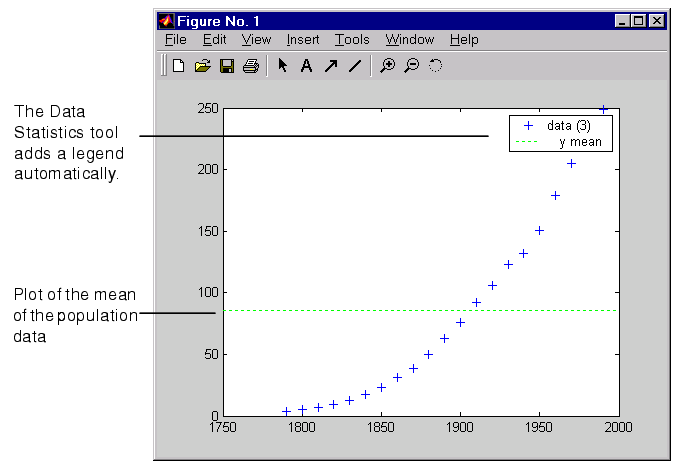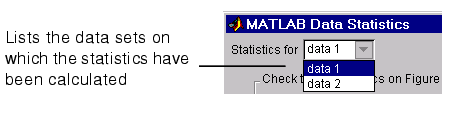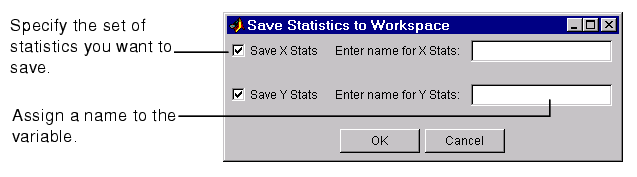GraphicsExample -- Plotting the Mean of a Data Set

To add a plot of the mean of a data set to a graph,

1. Plot your data. For example, use these commands to plot historical population data from the United States census.
• ```load census
plot(cdate,pop,'+')
```
2. Select the Data Statistics option from the figure window Tools menu.
1. The Data Statistics tool calculates basic statistics on the x-data and y-data of the plot in the graph and displays the results in a dialog box.1. Select the statistic you want to plot in your graph by clicking in the check box next to the value.
1. For example, to add a plot of the mean of the population data (y-data) to the graph, click in the check box next to the value (as shown in the figure). The Data Statistics tool adds the plot of the mean to the graph.Using a Legend with Data Statistics

When you activate the Data Statistics tool, it calculates statistics for the plotted data and automatically adds a legend to the graph, if the graph doesn't already have one.

When you add a plot of one or more statistics to the graph, the Data Statistics tool adds an entry in the legend for the new plot. The Data Statistics tool assigns the plotted statistic a descriptive name that identifies it in the legend. In the example, the plotted statistic has the name `y mean` in the legend.

Formatting Plots of Data Statistics

The Data Statistics tool uses color and line style to distinguish the plots of statistics from the other plots in a graph. However, as with any other plot in a graph, you can change these characteristics.

 Note    Do not edit the format of the plots of data statistics until you are finished adding them to a graph. If you edit a plot of data statistics, delete the plot, and then add it back, any formatting you did to the plotted statistics will be lost.

To modify the properties of a plotted statistic,

1. Enable plot editing mode in the figure window.
2. Double-click on the plot of the statistic. This starts the Property Editor, which provides access to properties of the line object used to plot the statistic.
1. You can also access a subset of these properties by right-clicking on the plot. This brings up the plot's context menu, which includes options for specifying line width, line style, and color.

1. Change the properties of the plot and click Apply.

Statistics Plotted by the Data Statistics Tool

 Note    You can only use the Data Statistics tool to generate statistics for two-dimensional data (vectors and matrices).

The following table lists the statistics calculated by the Data Statistics tool. The table includes the name of the MATLAB function used to calculate the statistic. For more information about these statistical functions, see Basic Data Analysis Functions in the MATLAB Math documentation.

 Statistic Description MATLAB Function Maximum Largest value in the data set `max` Minimum Smallest value in the data set `min` Mean Average of all the values in the data set `mean` Median Middle value in the data set `median` Range Interval between the lowest value and the highest value in the data set. The Data Statistic tool does not plot the range statistic. n/a Standard deviation A measure characterizing the amount of variation among the values in the data setNote: The Data Statistics tool uses two lines to plot the standard deviation in a graph. The lines represent the boundaries of one standard deviation on either side of the mean of the data set. `std`

Automatic Updating of Statistics

If you have the Data Statistics tool displayed and you change the x-data or y-data of a plot, the Data Statistics tool automatically regenerates the statistics for that plot.

Viewing Statistics for Multiple Plots

The Data Statistics tool calculates basic statistics for every 2-D plot in a graph, but displays the statistics for only one plot at time.

To view the statistics for a particular plot in a graph,

1. Click the Statistics for menu in the Data Statistics dialog box.1. This menu lists all the data sets plotted in the graph, identifying each data set by its tag. (A tag is a user-defined text string that can be associated with any graphics object.) For plots in the graph that do not have tags, the Data Statistics tool uses `data1` to identify the first plot, `data2` to identify the second plot, and so on.

1. Select a plot from the list. The Data Statistics tool updates the values displayed in the dialog box.

Saving Statistics to the MATLAB Workspace

To save the statistics generated by the Data Statistics tool to the MATLAB workspace, follow this procedure:

 Note    You must repeat this procedure for each plot in a graph containing multiple plots.

1. Click the Save to Workspace button.
2. In the Save Statistics to Workspace dialog box, specify the sets of statistics you want to save, x-data or y-data, and specify the names you want to assign to the variables in which the statistics will be stored.The Data Statistics tool saves each set of statistics in a structure. For example, if you save the set of statistics on the x-data in the census in the variable `census_dates`, the contents of the structure looks like this.

• ```census_dates =

min: 1790
max: 1990
mean: 1890
median: 1890
std: 62.0484
range: 200
```Adding Plots of Basic Statistics to Graphs Annotating Graphs© 1994-2005 The MathWorks, Inc.